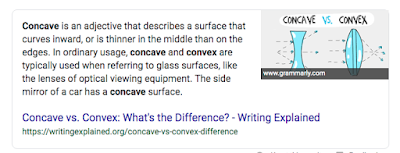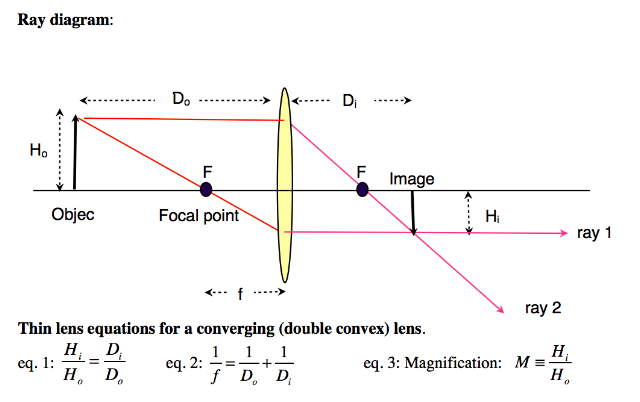## Wednesday, May 15, 2019

### Light and Optics: Introduction

Light

Light is one form of electromagnetic (EM) energy. Light is defined within a narrow range of electromagnetic frequencies which stimulate the receptors of the eye.  Included in light is the frequencies just beyond what humans can see. Infrared light has a slightly too low of a a frequency for humans to see. Ultraviolet light has a slightly too high frequency for humans to see.

All forms of electromagnetic energy are affected by interacting with matter. When light interacts with matter, it either reflects off of it,  refracts around it, or passes through.

One of the properties of light (and other EM types) is that it will propagate at different rates through different mediums. Though the difference is generally slight, it makes a difference.

Optics

Light, because it can be easily observed, provides many opportunities to EM radiation.Notice how the pencil seems to bend. This occurs because light travels at different rates through air and water (and glass).
The difference between the speed of light in a vacuum and the speed of light in air is very, very minimal (on a scale of X 108). However, the difference in speed between light in air and light in water water results in light bending at the waters surface.

The differences in speed also explain how lenses can reshape light. Glass can bend the light in one of two ways.

Focusing is the process of taking light that is moving in a straight line and concentrates it into a smaller area. The rays of light (particle model) are bent such that they converge (align into a tighter, smaller area).

Light can also be "un focused." Light that is traveling in a straight line can be dispersed into a wider area. The rays of light (particle model) are bent such that they diverge (spread out over a wider area).

Both lenses and mirrors can converge (focus) and diverge light. First… There are these three words you need to understand.From Google
Concave: A shape that has a recessed center relative to the outer edges. The center caves in.

Flat: The center and the edges are in the same plane. (A window)

Convex: A shape that has a protruding center relative to the outer edges. NOT like a cave (see above).

So, which types of lens and mirror do what?

• Focus occurs with convex lenses and concave mirrors.
• Dispersion occurs with concave lenses and convex mirrors.

STOP - NOTICE!Throughout the vast domain of information on optics, lenses, and light, there exists a variety of sets of variables that identify different measures. The "distance to the object from the lens," for example can be found as…
d… do… D… Do… u… o…
This variation is very frequent. This discussion reflects that with examples and images used from other sources.

Focal Length

Every lens has a focal length. That is the distance from the lens to the point where parallel light will be focused:An example of a convex mirror which spreads the light making objects appear smaller than they actually are.
Convex lenses have a positive focal length. The image appears some distance behind the lens. The lens is between the object and the image.

Concave lenses have a negative focal length. No image appears, but the focal point is in front of the lens, between the object and the lens.

Concave mirrors have a positive focal length. The image appears some distance behind the lens. The lens is between the object and the image.

Convex mirrors have a negative focal length. No image appears, but the focal point is in front of the lens, between the object and the lens.

A flat mirror and a flat piece of glass (window) work into this schema, falling between convex and concave. Imagine a lens that is convex. As the radius of the curve increases, it gets closer and closer to a flat piece of glass (a window). Likewise, a mirror can flatten out as well.

The object appears inside the mirror an equal distance, but behind the mirror. More on this to come!

Depending on the substance from which a lens is made as well as the geometry of the lens, there is a relationship between the distance from the object to the lens, o, the focal length of the lens f, and the distance from the lens to the projected image i.

Object ------ o ------ Lens ----- i -------Image

That relationship is:
1/o + 1/i = 1/f

Solving for f… (consult with a math teacher if you have questions!)

(i/i)(1/o) + (o/o)(1/i) = 1/f

(i/io) + (o/io) = 1/f

(i + o)/(i)(o)  = 1/f

f = (i)(o)/(i + o)

So, knowing i and o, we can easily find f:

EXAMPLE:

An object is 2 cm from a lens and the image appears 5 cm from the lens. Find the focal length.
f = (i)(o)/(i + o)
f = (2 • 4)/(2 + 4)
f = 8 / 6
f = 1.333 cm

An interesting property to examine is the relationship of image size to object size in consideration of where the object is located and where the image appears.Image from http://www.retremblay.net/PhyLifePart01/Individual_labs_files/lab10.pdf

The formula
Hi/Ho = Di/Do ( see eq. 1 above)
is not difficult to work with. Cross multiplying will quickly reduce the problem to a simple solution, for instance:

EXAMPLE:
Find the height of the image, Hi, when the object has a height of 3 cm and where the object is 8 cm from the lens and the image is 4 cm from the lens.

Hi/Ho = Di/Do
Hi/3cm = 8cm/4cm
cross multiply to get
(4cm)(Hi) = 24cm2
Hi = 6cm

Lastly, we can talk about magnification. How much bigger or smaller is the image compared to the object?.

This one is pretty easy!

M = hi/ho

So… divide…

EXAMPLE (without units, which would be a distance unit for hi and ho):

A lens has a magnification, M, of 1.25. I f the height of the object is 6, what is the height of the image?
M = hi/ho
1.25 = hi/6
(6)(1.25) = (hi/6)(6/1)
7.5 = hi

Conclusion

A very large portion of society has a daily encounter with optics: those who wear glasses or contacts and those take photos (camera or phone!)

While the need to calculate "things" rarely comes up, a fundamental understanding of lenses and light add to the daily life experience.

1 "propagates" is used to describe the motion of waves through a medium. Mechanical waves (such as sound) propagate by transferring energy through collision of molecules. Electromagnetic waves propagate differently and do not rely on matter as a medium. Once upon a time, scientists theorized that the universe was filled with something they called the aether that was responsible for the propagation of electromagnetic waves.